1596003660

## Gradient Boost Implementation = pytorch optimization + sklearn decision tree regressor

In order to understand the Gradient Boosting Algorithm, effort has been made to implement it from first principles using **pytorch **to perform the necessary optimizations (minimize loss function) and calculate the residuals (partial derivatives with respect to predictions) of the loss function and decision tree regressor from sklearn to create the regression decision trees.A line of trees

# Algorithm Outline

The outline of the algorithm can be seen in the following figure: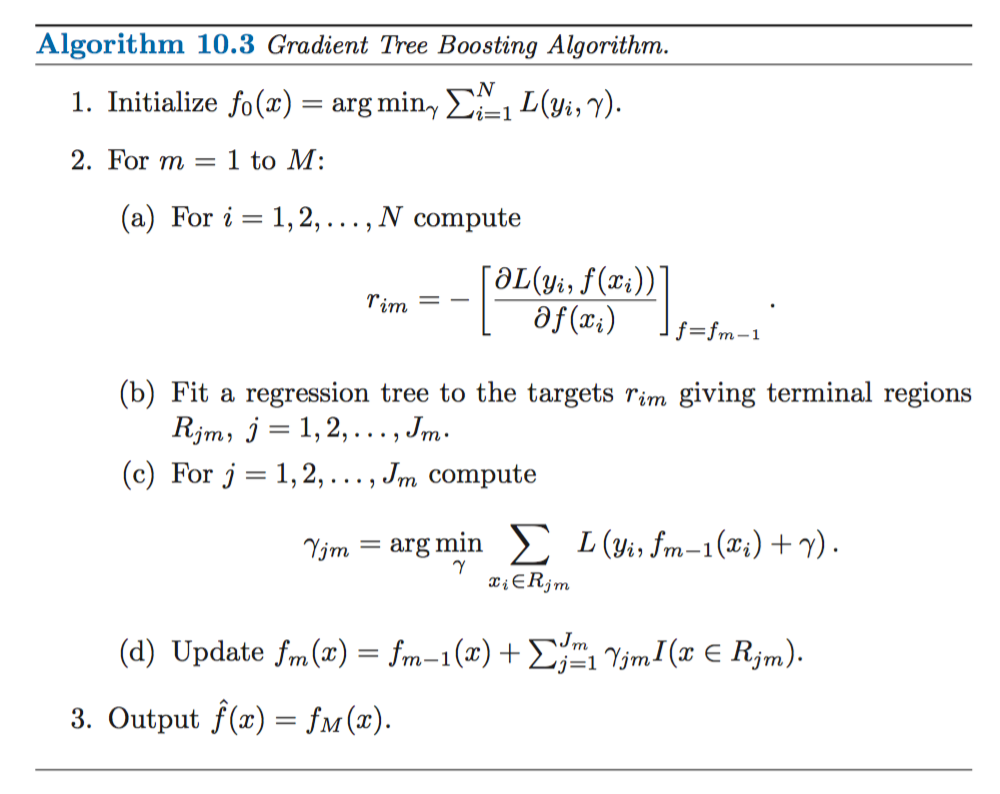Steps of the algorithm

We will try to implement this step by step and also try to understand why the steps the algorithm takes make sense along the way.

# Explanations + Code snippets

## Loss function minimization

What is crucial is that the algorithm tries to minimize a loss function, be it square distance in the case of regression or binary cross entropy in the case of classification.

The loss function parameters are the predictions we make for the training examples : L(prediction_for_train_point_1, prediction_for_train_pont_2, …., prediction_for_train_point_m).

## Buddha Community1596962880

The reign of the Gradient Boosters were almost complete in the land of tabular data. In most real world as well as competitions, there was hardly a solution which did not have at least one model from one of the gradient boosting algorithms. But as the machine learning community matured, and the machine learning applications started to be more in use, the need for uncertainty output became important. For classification, the output from Gradient Boosting was already in a form which lets you understand the confidence of the model in its prediction. But for regression problems, it wasn’t the case. The model spat out a number and told us this was its prediction. How do you get uncertainty estimates from a point prediction? And this problem was not just for Gradient Boosting algorithms. but was for almost all the major ML algorithms. This is the problem that the new kid on the block — NGBoost seeks to tackle.

If you’ve not read the previous parts of the series, I strongly advise you to read up, at least the first one where I talk about the Gradient Boosting algorithm, because I am going to take it as a given that you already know what Gradient Boosting is. I would also strongly suggest to read the VI(A) so that you have a better understanding of what Natural Gradients are.

The key innovation in NGBoost is the use of Natural Gradients instead of regular gradients in the boosting algorithm. And by adopting this probabilistic route, it models a full probability distribution over the outcome space, conditioned on the covariates.

The paper modularizes their approach into three components -

1. Base Learner
2. Parametric Distribution
3. Scoring Rule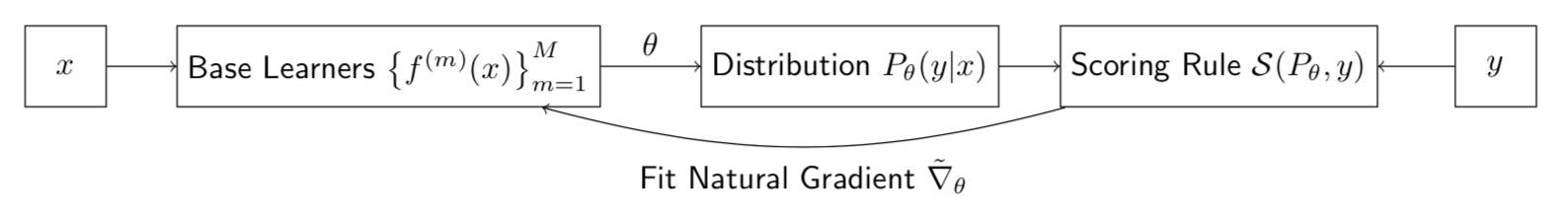## Base Learners

As in any boosting technique, there are base learners which are combined together to get a complete model. And the NGBoost doesn’t make any assumptions and states that the base learners can be any simple model. The implementation supports a Decision Tree and ridge Regression as base learners out of the box. But you can replace them with any other sci-kit learn style models just as easily.

## Parametric Distribution

Here, we are not training a model to predict the outcome as a point estimate, instead, we are predicting a full probability distribution. And every distribution is parametrized by a few parameters. For eg, the normal distribution is parametrized by its mean and standard deviation. You don’t need anything else to define a normal distribution. So, if we train the model to predict these parameters, instead of the point estimate, we will have a full probability distribution as the prediction.

## Scoring Rule

Any machine learning system works on a learning objective, and more often than not, it is the task of minimizing some loss. In point prediction, the predictions are compared with data with a loss function. Scoring rule is the analogue from the probabilistic regression world. The scoring rule compares the estimated probability distribution with the observed data.

A proper scoring rule, S, takes as input a forecasted probability distribution, P, and one observation y_(outcome)_, and assigns a score _S(P,y) _to the forecast such that the true distribution of the outcomes gets the best score in expectation.

The most commonly used proper scoring rule is the logarithmic score L, which, when minimized we get the MLE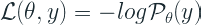which is nothing but the log likelihood that we have seen in so many places. And the scoring rule is parametrized by θ because that is what we are predicting as part of the machine learning model.

Another example is CRPS(Continuous Ranked Probability Score). While the logarithmic score or the log likelihood generalizes Mean Squared Error to a probabilistic space, CRPS does the same to Mean Absolute Error.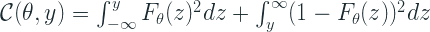In the last part of the series, we saw what Natural Gradient was. And in that discussion, we talked about KL Divergences, because traditionally, Natural Gradients were defined on the MLE scoring rule. But the paper proposes a generalization of the concept and provide a way to extend the concept to CRPS scoring rule as well. They generalized KL Divergence to a general Divergence and provided derivations for CRPS scoring rule.1593766336

## Introduction to the Gradient Boosting Algorithm

The Boosting Algorithm is one of the most powerful learning ideas introduced in the last twenty years. Gradient Boosting is an supervised machine learning algorithm used for classification and regression problems. It is an ensemble technique which uses multiple weak learners to produce a strong model for regression and classification.

# Intuition

Gradient Boosting relies on the intuition that the best possible next model , when combined with the previous models, minimizes the overall prediction errors. The key idea is to set the target outcomes from the previous models to the next model in order to minimize the errors. This is another boosting algorithm(few others are Adaboost, XGBoost etc.).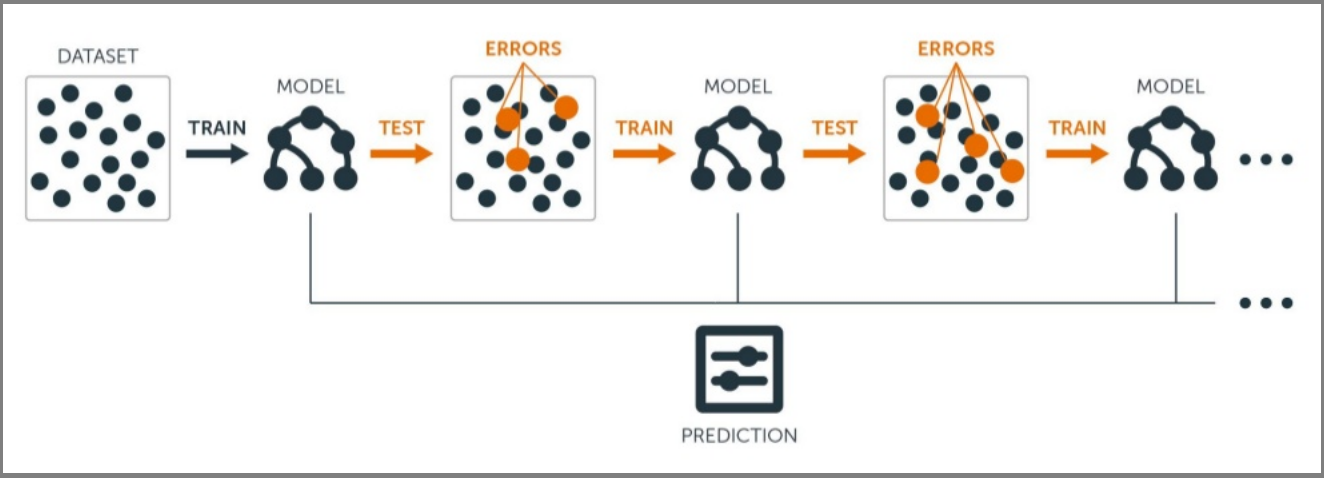1. A Loss Function to optimize.
2. A weak learner to make prediction(Generally Decision tree).
3. An additive model to add weak learners to minimize the loss function.

## 1. Loss Function

The loss function basically tells how my algorithm, models the data set.In simple terms it is difference between actual values and predicted values.

Regression Loss functions:

1. L1 loss or Mean Absolute Errors (MAE)
2. L2 Loss or Mean Square Error(MSE)

Binary Classification Loss Functions:

1. Binary Cross Entropy Loss
2. Hinge Loss

A gradient descent procedure is used to minimize the loss when adding trees.

## 2. Weak Learner

Weak learners are the models which is used sequentially to reduce the error generated from the previous models and to return a strong model on the end.

Decision trees are used as weak learner in gradient boosting algorithm.

In gradient boosting, decision trees are added one at a time (in sequence), and existing trees in the model are not changed.

# Understanding Gradient Boosting Step by Step :

This is our data set. Here Age, Sft., Location is independent variables and Price is dependent variable or Target variable.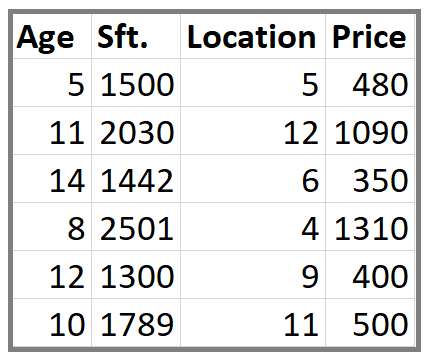Step 1: Calculate the average/mean of the target variable.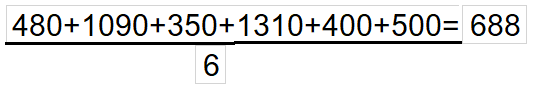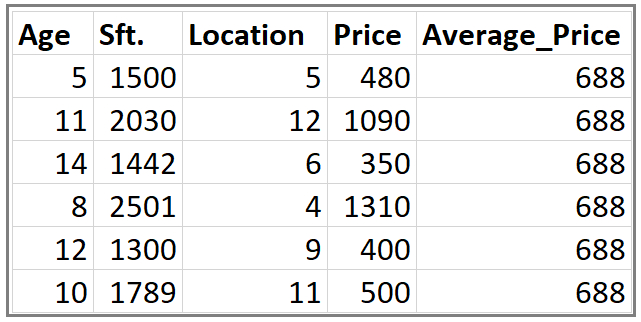Step 2: Calculate the residuals for each sample.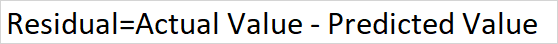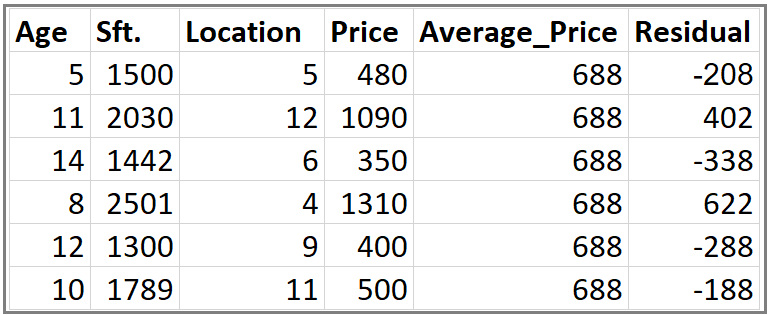**Step 3: **Construct a decision tree. We build a tree with the goal of predicting the Residuals.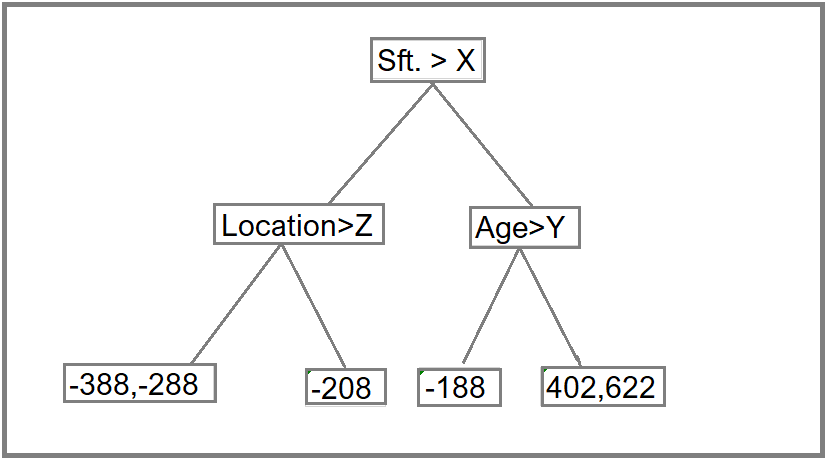In the event if there are more residuals then leaf nodes(here its 6 residuals),some residuals will end up inside the same leaf. When this happens, we compute their average and place that inside the leaf.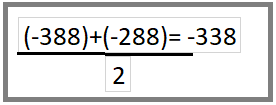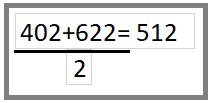After this tree become like this.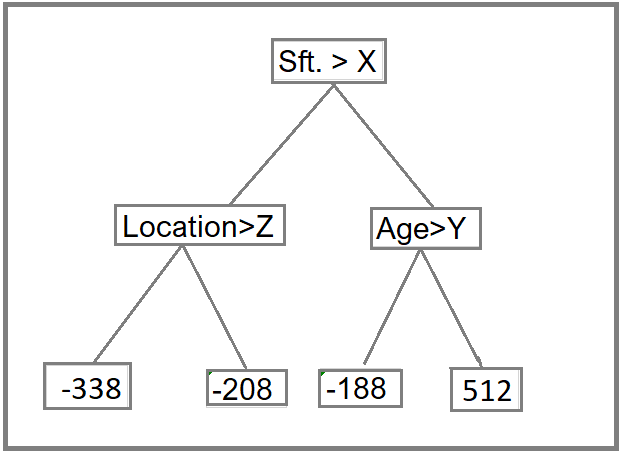Step 4: Predict the target label using all the trees within the ensemble.

Each sample passes through the decision nodes of the newly formed tree until it reaches a given lead. The residual in the said leaf is used to predict the house price.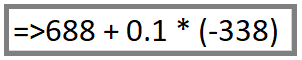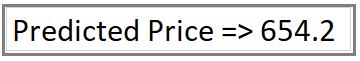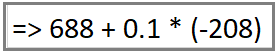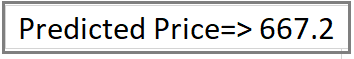Calculation above for Residual value (-338) and (-208) in Step 2

Same way we will calculate the Predicted Price for other values

Note: We have initially taken 0.1 as learning rate.

Step 5 : Compute the new residuals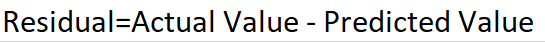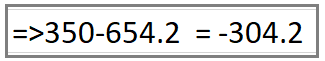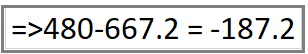When Price is 350 and 480 Respectively.1599348960

## What is Boosting?

Boosting is a very popular ensemble technique in which we combine many weak learners to transform them into a strong learner. Boosting is a sequential operation in which we build weak learners in series which are dependent on each other in a progressive manner i.e weak learner m depends on the output of weak learner m-1. The weak learners used in boosting have high bias and low variance. In nutshell boosting can be explained as boosting = weak learners + additive combing.

Gradient boosting is a machine learning technique for regression and classification problems, which produces a prediction model in the form of an ensemble of weak prediction models, typically decision trees (Wikipedia definition

## Algorithm steps: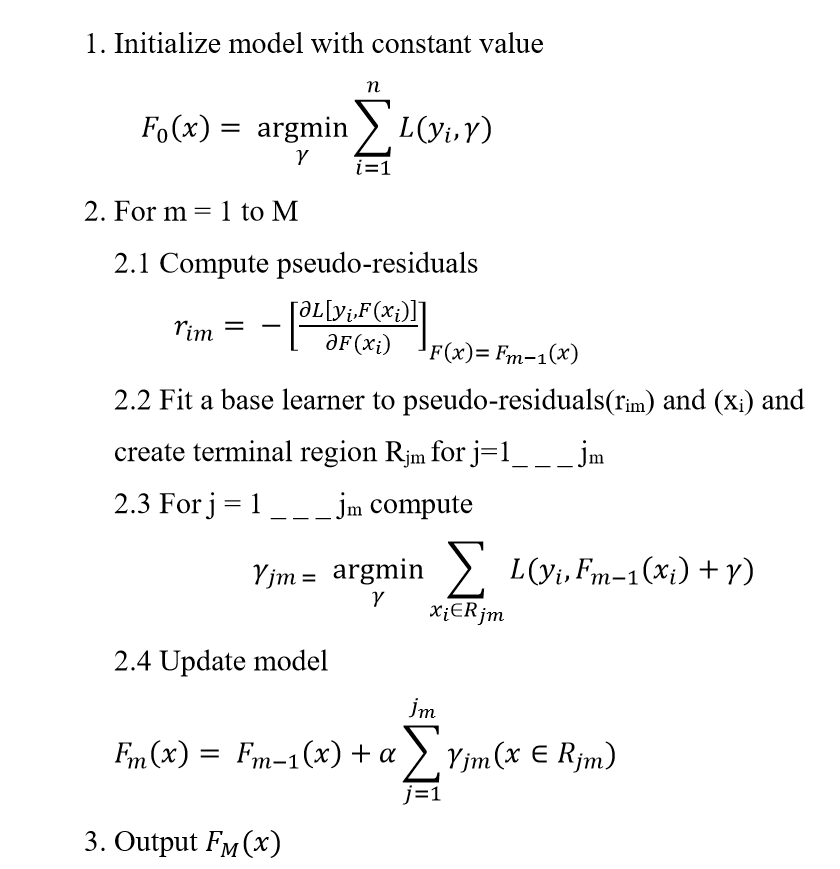1596003660

## Gradient Boost Implementation = pytorch optimization + sklearn decision tree regressor

In order to understand the Gradient Boosting Algorithm, effort has been made to implement it from first principles using **pytorch **to perform the necessary optimizations (minimize loss function) and calculate the residuals (partial derivatives with respect to predictions) of the loss function and decision tree regressor from sklearn to create the regression decision trees.A line of trees

# Algorithm Outline

The outline of the algorithm can be seen in the following figure:Steps of the algorithm

We will try to implement this step by step and also try to understand why the steps the algorithm takes make sense along the way.

# Explanations + Code snippets

## Loss function minimization

What is crucial is that the algorithm tries to minimize a loss function, be it square distance in the case of regression or binary cross entropy in the case of classification.

The loss function parameters are the predictions we make for the training examples : L(prediction_for_train_point_1, prediction_for_train_pont_2, …., prediction_for_train_point_m).1602939600

## Fast Gradient Boosting with CatBoost

In gradient boosting, predictions are made from an ensemble of weak learners. Unlike a random forest that creates a decision tree for each sample, in gradient boosting, trees are created one after the other. Previous trees in the model are not altered. Results from the previous tree are used to improve the next one. In this piece, we’ll take a closer look at a gradient boosting library called CatBoost.

CatBoost is a depth-wise gradient boosting library developed by Yandex. It uses oblivious decision trees to grow a balanced tree. The same features are used to make left and right splits for each level of the tree.source

As compared to classic trees, the oblivious trees are more efficient to implement on CPU and are simple to fit.

#overviews #catboost #gradient boosting #machine learning #python